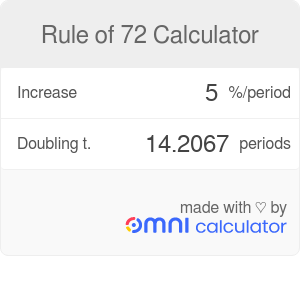Increase
%
/period
Doubling t.
periods

# Rule of 72 Calculator

By Mateusz Mucha

Rule of 72 calculator tells you how much time it takes for something to double given a certain level of constant growth rate. The term "doubling time" can be used interchangeably - our tool could also be called "doubling time calculator", because these two terms are synonymous. We've also included a nifty trick that lets you easily do it manually, see below in the "what is rule of 72?" section. This is actually a special kind of compound interest, where you are trying to find how long it will take for something (for example your investment) to increase by 100%. It's a fairly narrow case, but it is actually quite common.

## What is the rule of 72?

The rule states that in order to get the estimated doubling time, simply divide `72` by the percentage growth over a single period. For example, if something grows by `5%` per minute, then it will double in roughly `72 / 5 = 14.4 minutes`. The actual number is closer to 14.2067, but this example should give you an idea of the difference between the rule of a thumb method and the true value.

## Things to consider when using the doubling time calculator

• It gives you exact number (well, it's usually rounded), while a "manual" rule of 72 calculation gives you a ballpark figure.
• Rule of 72 calculator is unit-agnostic. It doesn't matter if we're talking about seconds, minutes, days or dog years.
• It works just as well in reverse. For example, if you want to double your web traffic in `52 weeks`, you know it needs to grow by `1.3419%` per week.
• It assumes that the percentage growth is exactly the same each period, so it works very well with simple interest (when it basically boils down to compound annual growth rate). Please be aware, however, that percentages (such as your web traffic's increase) often fluctuate and are never stable, so known the limitations of this calculation.
Mateusz Mucha

## Get the widget!

Rule of 72 Calculator can be embedded on your website to enrich the content you wrote and make it easier for your visitors to understand your message.

It is free, awesome and will keep people coming back!